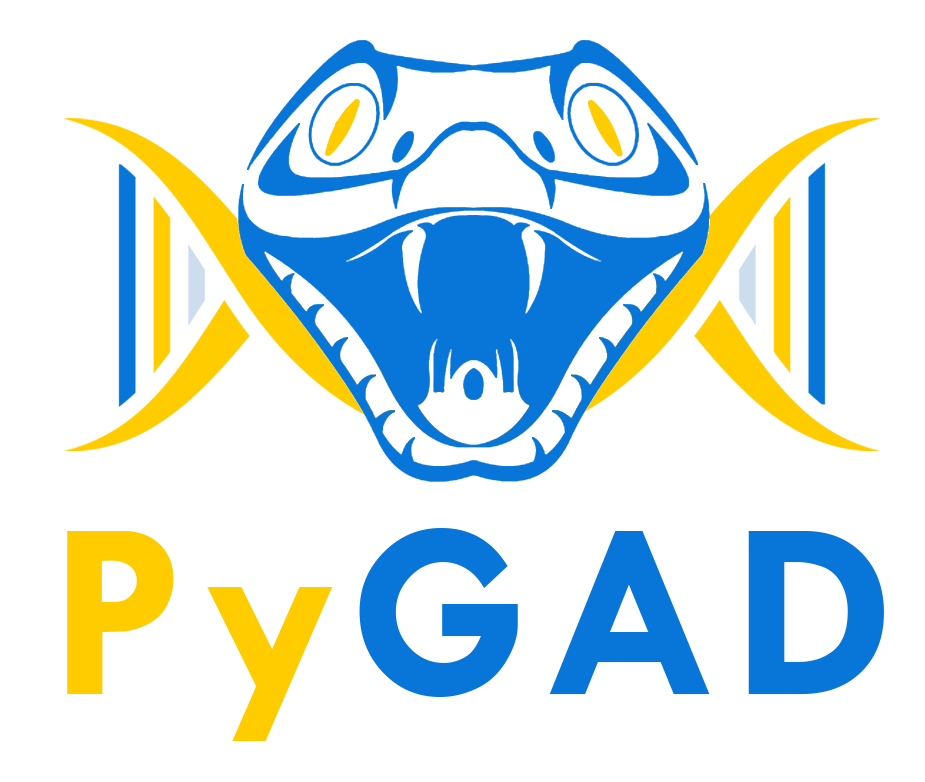PyGAD is an open-source Python library for building the genetic algorithm and optimizing machine learning algorithms. It works with Keras and PyTorch.

PyGAD supports different types of crossover, mutation, and parent selection operators. PyGAD allows different types of problems to be optimized using the genetic algorithm by customizing the fitness function.Logo designed byAsmaa Kabil

Besides building the genetic algorithm, it builds and optimizes machine learning algorithms. Currently, PyGAD supports building and training (using genetic algorithm) artificial neural networks for classification problems.

Donation & Support¶

You can donate to PyGAD via:

Installation¶

Install PyGAD with the following command:

PyGAD is developed in Python 3.7.3 and depends on NumPy for creating and manipulating arrays and Matplotlib for creating figures. The exact NumPy version used in developing PyGAD is 1.16.4. For Matplotlib, the version is 3.1.0.

Quick Start¶

To get started with PyGAD, simply import it.

Using PyGAD, a wide range of problems can be optimized. A quick and simple problem to be optimized using the PyGAD is finding the best set of weights that satisfy the following function:

y = f(w1:w6) = w1x1 + w2x2 + w3x3 + w4x4 + w5x5 + 6wx6
where (x1,x2,x3,x4,x5,x6)=(4,-2,3.5,5,-11,-4.7) and y=44

The first step is to prepare the inputs and the outputs of this equation.

function_inputs = [4,-2,3.5,5,-11,-4.7]
desired_output = 44

A very important step is to implement the fitness function that will be used for calculating the fitness value for each solution. Here is one.

def fitness_func(solution, solution_idx):
output = numpy.sum(solution*function_inputs)
fitness = 1.0 / numpy.abs(output - desired_output)
return fitness

Next is to prepare the parameters of PyGAD. Here is an example for a set of parameters.

fitness_function = fitness_func

num_generations = 50
num_parents_mating = 4

sol_per_pop = 8
num_genes = len(function_inputs)

init_range_low = -2
init_range_high = 5

parent_selection_type = "sss"
keep_parents = 1

crossover_type = "single_point"

mutation_type = "random"
mutation_percent_genes = 10

After the parameters are prepared, an instance of the pygad.GA class is created.

num_parents_mating=num_parents_mating,
fitness_func=fitness_function,
sol_per_pop=sol_per_pop,
num_genes=num_genes,
init_range_low=init_range_low,
init_range_high=init_range_high,
parent_selection_type=parent_selection_type,
keep_parents=keep_parents,
crossover_type=crossover_type,
mutation_type=mutation_type,
mutation_percent_genes=mutation_percent_genes)

After creating the instance, the run() method is called to start the optimization.

ga_instance.run()

After the run() method completes, information about the best solution found by PyGAD can be accessed.

solution, solution_fitness, solution_idx = ga_instance.best_solution()
print("Parameters of the best solution : {solution}".format(solution=solution))
print("Fitness value of the best solution = {solution_fitness}".format(solution_fitness=solution_fitness))

prediction = numpy.sum(numpy.array(function_inputs)*solution)
print("Predicted output based on the best solution : {prediction}".format(prediction=prediction))
Parameters of the best solution : [3.92692328 -0.11554946 2.39873381 3.29579039 -0.74091476 1.05468517]
Fitness value of the best solution = 157.37320042925006
Predicted output based on the best solution : 44.00635432206546

1. The main module has the same name as the library which is pygad that builds the genetic algorithm.
2. The nn module builds artificial neural networks.
3. The gann module optimizes neural networks (for classification and regression) using the genetic algorithm.
4. The cnn module builds convolutional neural networks.
5. The gacnn module optimizes convolutional neural networks using the genetic algorithm.
6. The kerasga module to train Keras models using the genetic algorithm.
7. The torchga module to train PyTorch models using the genetic algorithm.

The documentation discusses each of these modules.

If you used PyGAD, please consider citing its paper with the following details:

title={PyGAD: An Intuitive Genetic Algorithm Python Library},